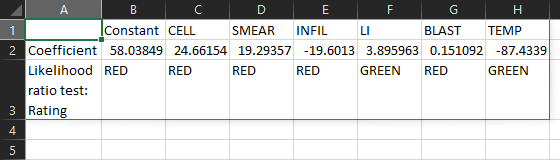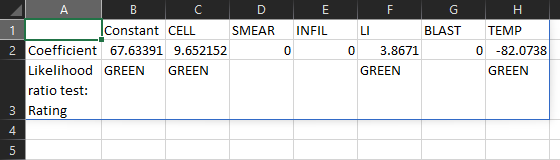How to perform logistic regression in Excel using only a formula

Demonstrations

Logistic regression is a process for modelling the probability of a binary outcome in terms of explanatory factors using a logistic function. It can be used to model the probability of a risk event occurring, such as credit default and insurance fraud.

QRS.LOGISTIC.REGRESSION

QRS Toolbox for Excel includes the QRS.LOGISTIC.REGRESSION function for performing logistic regression using nothing more than a formula. The function includes options to return the same results as more expensive commercial products.

In the workbook:

• Cells A7–A33 contain identifiers for 27 leukemia patients.
• Cells B7–B33 contain ones if remission occurred and zeros otherwise.
• Cells C7–H33 contain factors that potentially explain the occurrence of remission.
• Cells C6–H6 contain shortened names of the factors.

Constant and coefficients

To perform a logistic regression between the occurrence of remission and the given factors, enter the formula =QRS.LOGISTIC.REGRESSION(C7:H33, B7:B33) in cell A1. The result contains 7 numbers. The first number is the regression constant. The remaining 6 numbers are the coefficients of the factors.

To improve the presentation of the result, add "LABELS", TRUE and "NAMES", C6:H6 to the formula. The result now contains row labels and column headers.

Significance tests

To determine the statistical significance of the factors, add "LRTEST", "RAG" to the formula. The result now contains red/amber/green ratings that summarize the likelihood ratio test for each factor.

The LI factor has a green rating. The other factors have red ratings. A green/amber rating means a factor is significant at the 5%/10% significance level. A red rating means a factor is not significant at the 10% significance level.

Please read the documentation to learn how to return the test statistic and p-value of the likelihood ratio test, as well as the corresponding results of the Wald test.

Significance levels=QRS.LOGISTIC.REGRESSION(C7:H33, B7:B33, "LABELS", TRUE, "NAMES", C6:H6, "LRTEST", "RAG", "PGREEN", 0.3, "PRED", 0.35)

To change the significance levels from the default values of 5% and 10% to, say, 30% and 35%, add "PGREEN", 0.3 and "PRED", 0.35 to the formula. The TEMP factor now has a green rating too.

Manual factor selection

To manually select only the LI and TEMP factors, enter 0, 0, 0, 1, 0, 1 in cells C5–H5 and add "MASK", C5:H5 to the formula. The excluded factors now have coefficients equal to zero.

Automatic factor selection=QRS.LOGISTIC.REGRESSION(C7:H33, B7:B33, "LABELS", TRUE, "NAMES", C6:H6, "LRTEST", "RAG", "PGREEN", 0.3, "PRED", 0.35, "METHOD", "STEPWISE")

To automatically select factors using stepwise selection, remove "MASK", C5:H5 and add "METHOD", "STEPWISE" to the formula. The automatically selected factors are CELL, LI, and TEMP.

Please read the documentation to learn how to use forward selection or backward elimination instead, and how to control the significance levels for factor selection.

QRS.LOGISTIC.MODEL

You can calculate the probability modelled by a logistic regression in Excel using the QRS.LOGISTIC.MODEL function.

Continuing from the example above, to calculate the probability of remission, enter the formula =QRS.LOGISTIC.MODEL(B\$2:H\$2, C7:H7) in cell I7, and copy the formula across cells I8–I33.

The probability of remission for Patient 01 is 72%. The probability for Patient 02 is 58%. The probability for Patient 03 is 10%, and so on.

Final remarks

If you find QRS.LOGISTIC.REGRESSION useful and would like to use it beyond your free trial period, you may purchase the right to use it indefinitely for as little as USD 29.00.

Get from AppSourceGet from Excel

1. Click Insert > Get Add-ins
2. Search for QRS Toolbox